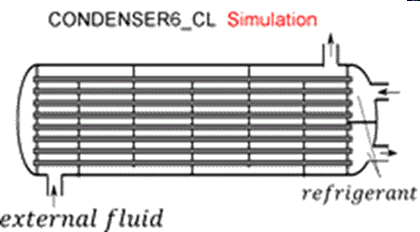Contents - index

Condenser6_CLCondenser6_CL determines the outlet state of a condenser cooled by an external fluid given the inlet states of the external fluid and refrigerant and the heat transfer surface area and heat transfer coefficients.  The refrigerant is expected to enter the condenser in a superheated state.   Thermal energy is rejected to an external fluid, which may be a pure liquid such as water or a brine.  It is necessary to provide the total heat exchanger area, the heat transfer coefficients on the external fluid side of the heat exchanger and for the superheated, saturated, and subcooled sections on the refrigerant side.  The heat transfer coefficients can be determined using the functions in the Heat Transfer Library, as illustrated in the expample provided for Condenser5_CL.  This library file is intended to be used to determine the outlet states for a condenser of fixed design.  Use Condenser5_CL to determine the required heat exchanger area at design conditions.

Inputs:

R\$:   name of the refrigerant

m_dot_R:  refrigerant flow rate (kg/s, lbm/hr)

h_R_in:  specific enthalpy of entering refrigerant (J/kg, kJ/kg, Btu/lbm)

P_R:  refrigerant pressure (Pa, kPa, bar, MPa, psia, atm)

Config\$: a string or string variable that must be set to either 'parallelflow' or 'counterflow'

m_dot_fluid:  mass flow rate of the external fluid (kg/s, lbm/hr)

T_fluid_in:  external fluid inlet temperature  (C, K, F, R)

cp_fluid:  specific heat of external fluid (J/kg-K, kJ/kg-K, Btu/lbm_R)

h_fluid:  effective outside (external fluid) heat transfer coefficient per unit refrigerant tube area (W/m^2-K, Btu/hr-ft^2-R)

A_fluid\A_R:  ratio of effective heat transfer area on the external fluid side to the heat transfer area for the refrigerant*

h_R_sh:  heat transfer coefficient for refrigerant in the superheated regime  (W/m^2-K, Btu/hr-ft^2-R)

h_R_sat:  average heat transfer coefficient for refrigerant in the saturated regime  (W/m^2-K, Btu/hr-ft^2-R)

h_R_sc:  average heat transfer coefficient for refrigerant in the subcooled regime  (W/m^2-K, Btu/hr-ft^2-R)

A_R: heat transfer area on the refrigerant side (m^2, ft^2)

Outputs:

Q_dot:  overall heat transfer rate (W, kW, Btu/hr)

h_R_out refrigerant outlet specific enthalpy (J/kg, kJ/kg, Btu/lbm)

T_fluid_out:  outlet temperature of the air  (C, K, F, R)

f_sh: fraction of the surface area used for the desuperheating

f_sc: fraction of the surface area used for subcooling

*A_fluid\A_R is the ratio of the product of the overall fin efficiency and total (finned and unfinned) area on the external fluid side to the total surface area inside the refrigerant flow passages.  For unfinned surfaces, A_fluid\A_R is the ratio of the outer surface to inner surface areas.

Notes:

In actual operation, the heat exchanger type may shell-in-tube, plate or other type.  However, Config\$ may only be set to 'Parallelflow' or 'Counterflow'.  These two options provide upper and lower bounds on the required heat transer area.

Example:

\$unitSystem SI C kPa kJ mass

\$TabStops 3 in

\$VarInfo cp_fluid units=kJ/kg-K

\$VarInfo h_R_in units=kJ/kg

\$VarInfo h_R_out units=kJ/kg

\$VarInfo Q_dot units=kW

\$VarInfo T_fluid_out units=C

R\$='R134a"refrigerant"

P_R=1 [MPa]*convert(MPa,kPa)  "refrigerant pressure"

m_dot_R=0.075 [kg/s]  "refrigerant mass flow rate"

T_R_in=45 [C]  "inlet refrigerant temperature"

h_R_in=enthalpy(R\$,T=T_R_in,P=P_R)  "inlet refrigerant specific enthalpy"

Fluid\$='EG' "ethylene glycol-water is the external fluid"

Conc=20 [%] "concentration of the ethylene glycol - water solution"

T_fluid_in=20 [C]  "inlet temperature of external fluid"

Config\$='counterflow{parallelflow} "heat exchanger configuration;  counterflow and parallelflow bound the problem"

m_dot_fluid=0.5 [kg/s] "mass flow rate of external fluid"

cp_fluid=cp(EG,T=T_fluid_in,C=Conc) "specific heat of external fluid"

h_fluid=620 [W/m^2-K]  "convection coefficient for external fluid side.  See h_fluid1 calculation below"

A_fluid\A_R=1.22 [-]  "ratio of area on external fluid side to refrigerant side.  See A_fluid\A_R1 calculation"

h_R_sh=815 [W/m^2-K]  "convection coefficient for superheated R134a.  See h_H_sh calculation"

h_R_sat=3825 [W/m^2-K]  "convection coefficient for saturated R134a.  See h_cond calculation"

h_R_SC=970 [W/m^2-K]  "heat transfer coefficient in subcooled section.  See h_H_sc calculation"

A_R=1.33 [m^2] "total area required"

Call condenser6_cl(R\$, m_dot_R, h_R_in, P_R, Config\$, m_dot_fluid, cp_fluid, T_fluid_in,  h_fluid, A_fluid\A_R, h_R_sh, h_R_sat, h_R_sc, A_R : Q_dot, h_R_out, T_fluid_out, f_sh, f_sc)

{Solution

A_fluid\A_R=1.22 [-]

A_R=1.33 [m^2]

Conc=20 [%]

Config\$='counterflow'

cp_fluid=3.896 [kJ/kg-K]

Fluid\$='EG'

f_sc=0.02195 [-]

f_sh=0.05847 [-]

h_fluid=620 [W/m^2-K]

h_R_in=277.3 [kJ/kg]

h_R_out=104.3 [kJ/kg]

h_R_sat=3825 [W/m^2-K]

h_R_SC=970 [W/m^2-K]

h_R_sh=815 [W/m^2-K]

m_dot_fluid=0.5 [kg/s]

m_dot_R=0.075 [kg/s]

P_R=1000 [kPa]

Q_dot=12.97 [kW]

R\$='R134A'

T_fluid_in=20 [C]

T_fluid_out=26.66 [C]

T_R_in=45 [C]

}